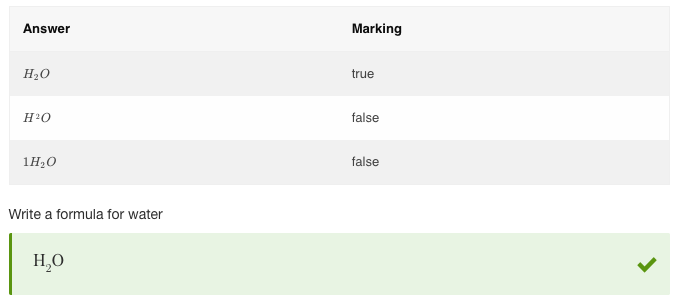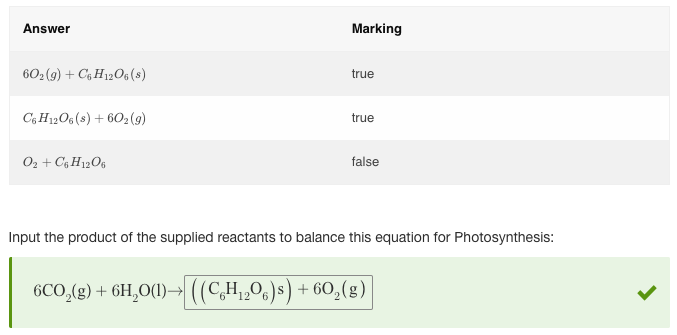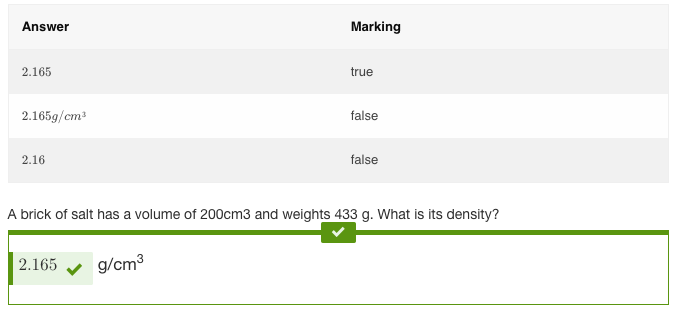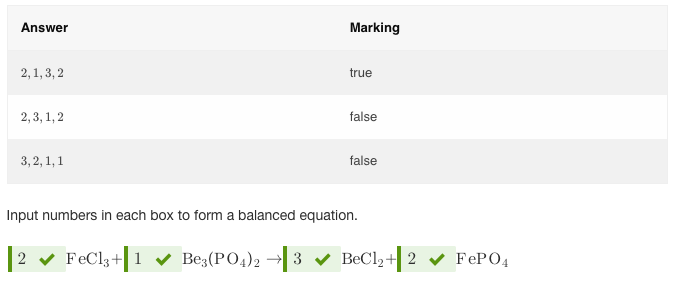# equivLiteral Scoring Method

## Overview

The equivLiteral method checks if a chemistry expression is literally equal to the one set in validation. Both expressions should have the same form, order, elements, and values. Student responses that are equal to the response set in validation, but are in a form other than the one specified by authors, will return false. Use equivLiteral when you want to specify a particular form of the chemical expression as the correct answer.

• Ignore order
Ignores the order of expressions. E.g. both x+1 and 1+x will be considered correct. If this is not enabled, equivLiteral will not accept the correct response specified by the Author in a different order, even if it is an acceptable answer.

• Inverse result
Enabling this means that the value specified in the Value field will not be accepted as the correct answer. It is a useful way of excluding very specific answers from validation. Use case: Students are asked to enter something symbolically equivalent to `(x+2)^2`, however you don't want them to enter the same expression and score points for such response. In this case, adding an additional scoring method equivLiteral and enabling Inverse Result will exclude (x+2)^2 from correct response options. See examples in the Combining Methods section below.

## Examples

Example 1 - Basic equivLiteral scoring

Use equivLiteral scoring for chemistry questions where there is only one possible correct response.Figure 1: Basic equivLiteral scoring

Example 2 - Ignore order

In questions where students are asked to balance chemistry equations, use equivLiteral scoring with Ignore order enabled. This will ensure the order of the elements will not affect validation.Figure 2: Ignore order

Example 3 - Cloze chemistry

In this example, the students are asked to enter the correct answer in the response box. The response should match the value set in validation.Figure 3: Cloze chemistry

Example 4 - Cloze Chemistry - Multiple responses

This is a cloze question with 4 response boxes. The order of responses cannot be changed.Figure 4: Cloze chemistry - multiple responses